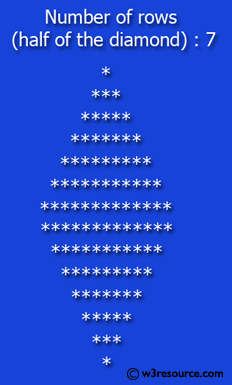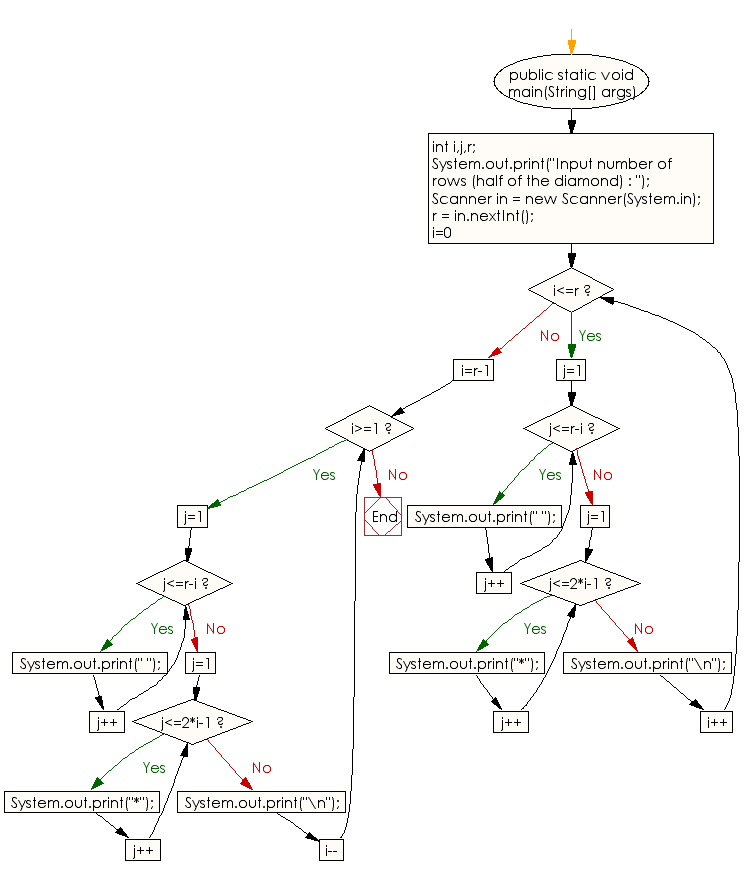﻿ Java exercises: Display the pattern like a diamond - w3resource# Java Conditional Statement Exercises: Display the pattern like a diamond

## Java Conditional Statement: Exercise-21 with Solution

Write a program in Java to display the pattern like a diamond.

Test Data
Input number of rows (half of the diamond) :7

Pictorial Presentation:Sample Solution:

Java Code:

``````import java.util.Scanner;
public class Exercise21 {

public static void main(String[] args)

{
int i,j,r;
System.out.print("Input number of rows (half of the diamond) : ");
Scanner in = new Scanner(System.in);
r = in.nextInt();
for(i=0;i<=r;i++)
{
for(j=1;j<=r-i;j++)
System.out.print(" ");
for(j=1;j<=2*i-1;j++)
System.out.print("*");
System.out.print("\n");
}

for(i=r-1;i>=1;i--)
{
for(j=1;j<=r-i;j++)
System.out.print(" ");
for(j=1;j<=2*i-1;j++)
System.out.print("*");
System.out.print("\n");
}

}
}
```
```

Sample Output:

```Input number of rows (half of the diamond) : 7
*
***
*****
*******
*********
***********
*************
***********
*********
*******
*****
***
*

```

Flowchart:Java Code Editor: